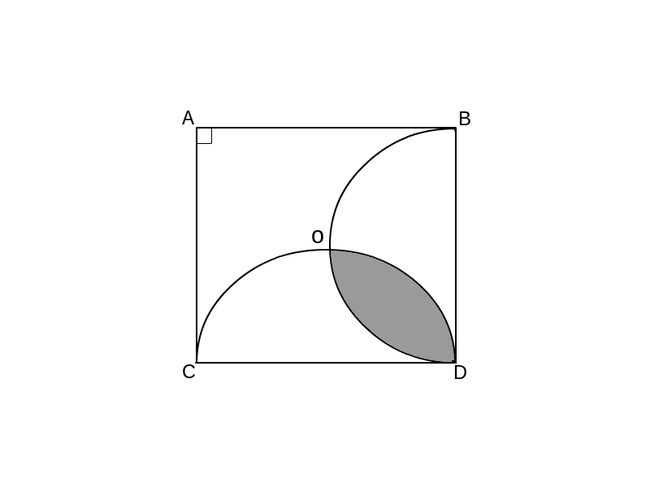# A geometry problem by Saimir Sulaj

Geometry Level 3Above shows a unit square $ABCD$ such that both semi-circles passing through the centre of the square, $O$. Find the area of the shaded region. Answer to 3 significant figures.

×